# 8.3 Vector Fields and Integral Curves

To consider feedback motion plans over continuous state spaces, including configuration spaces, we will need to define a vector field and the trajectory that is obtained by integrating the vector field from an initial point. A vector field is ideal for characterizing a feedback plan over a continuous state space. It can be viewed as providing the continuous-space analog to the feedback plans on grids, as shown in Figure 8.2b.

This section presents two alternative presentations of the background mathematical concepts. Section 8.3.1 assumes that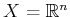, which leads to definitions that appear very similar to those you may have learned in basic calculus and differential equations courses. Section 8.3.2 covers the more general case of vector fields on manifolds. This requires significantly more technical concepts and builds on the manifold definitions of Section 4.1.2.

Some readers may have already had some background in differentiable manifolds. If, however, you are seeing it for the first time, then it may be difficult to comprehend on the first reading. In addition to rereading, here are two other suggestions. First, try studying background material on this subject, which is suggested at the end of the chapter. Second, disregard the manifold technicalities in the subsequent sections and pretend that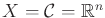. Nearly everything will make sense without the additional technicalities. Imagine that a manifold is defined as a cube,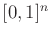, with some sides identified, as in Section 4.1.2. The concepts that were presented for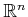can be applied everywhere except at the boundary of the cube. For example, if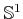is defined as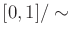, and a function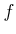is defined on, how can we define the derivative at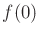? The technical definitions of Section 8.3.2 fix this problem. Sometimes, the technicalities can be avoided in practice by cleverly handling the identification points.

Subsections
Steven M LaValle 2020-08-14